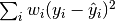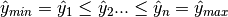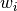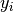This documentation is for scikit-learn version 0.15-gitOther versions

If you use the software, please consider citing scikit-learn.

# 1.14. Isotonic regression¶

The class IsotonicRegression fits a non-decreasing function to data. It solves the following problem:

minimizesubject towhere eachis strictly positive and eachis an arbitrary real number. It yields the vector which is composed of non-decreasing elements the closest in terms of mean squared error. In practice this list of elements forms a function that is piecewise linear.